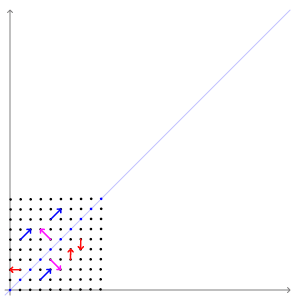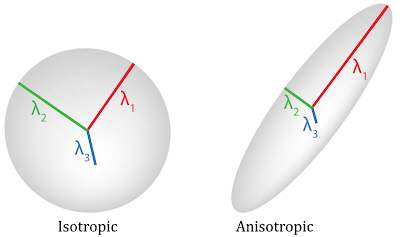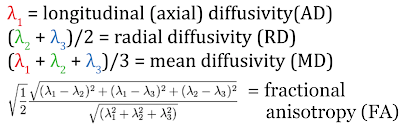# The diffusion tensor, and its relation to FA, MD, AD and RD Thursday, October 08, 2015 Do Tromp 0 Comments

In DTI 101 we described how diffusion tensor imaging estimates brain microstructure by modeling diffusion of water in the brain. We briefly discuss that this is done by using ellipsoid or ball shaped tensors. This leads to the question; what is a tensor?

"Tensors are simply mathematical objects that can be used to describe physical properties, just like scalars and vectors." - University of Cambridge
Or, more specifically as described by Kahn Academy:

In the scanner the diffusion tensor is measured by imaging the diffusion in individual gradient directions, like in the image below. If you are interested a more in depth mathematical explanation of this principle is provided in our post here.Example work out for 6 gradient directions, from diffusion image to tensor
Once the water diffusion is measured there are a number of ways you can quantify the shape of the tensors in each voxel. In DTI there are 4 measures that are most commonly used; fractional anisotropy, mean diffusivity, axial diffusivity and radial diffusivity. These measure directly relate to the value of the three main eigenvalues of the tensor, indicated in the figure below with lambda 1lambda 2 and lambda 3. What is an eigenvalue of a tensor? It is the value of the displacement/diffusion for each specific vector. Or, as defined by wikipedia:

"If a two-dimensional space is visualized as a rubber sheet, a linear map with two eigenvectors and associated eigenvalues λ1 and λ2 may be envisioned as stretching/compressing the sheet simultaneously along the two directions of the eigenvectors with the factors given by the eigenvalues." - WikipediaExample of the influence of the transformation (or in our case diffusion) on the eigenvalues
There are roughly two archetypical examples of diffusion tensors. On the left you see the example where the tensor has roughly equal eigenvalues for each main vector, thus showing an isotropic diffusion profile. On the right an example of diffusion primarily in one direction, thus demonstrating an anisotropic diffusion profile.Examples of tensor shapes
Characterization of each eigenvalue in the tensor and combinations of them help constitute the main diffusion measures. Specifically, in axial diffusivity (AD) we only quantify the value of lambda 1. While in radial diffusivity (RD) we take the average of lambda 2 and lambda 3. Mean diffusivity (MD) provides an average of all three, lambda 1, lambda 2, and lambda 3, this measure is sometimes also referred to as trace (TR), which is the sum of lambda 1lambda 2 and lambda 3. And finally fractional anisotropy (FA) provides the relative difference between the largest eigenvalue as compared to the others; it quantifies the fraction of diffusion that is anisotropic. The exact mathematical relations are shown below:Relation of diffusivity measures to the eigenvalues of the tensor
When you plot the value of each a diffusivity measure in every voxel you can get scalar maps of the quantification of the local tensors. In the image below you see representative examples of each measure. When you look at the MD map it becomes apparent that the mean diffusivity measure is specifically sensitive to cerebral spinal fluid (CSF), which has high values of average diffusion. In voxels with much CSF mean diffusivity is high, and values are thus bright. While AD is only sensitive to diffusion in the longest eigenvalue. Here you see that highly organized structures like white matter pathways are brights. In addition large open cavities like ventricles have general high levels of diffusion which translates to high lambda 1 values. Then RD represents the two shortest eigenvalues and shows dark values in highly organized and dense structures like white matter pathways, intermediate values in gray matter, and high values in regions with CSF. Finally, FA plots the relative length of lambda 1, compared to lambda 2 and lambda 3. This leads to selective brightness in white matter, but not gray matter or CSF.Examples of each diffusivity measure, FA, MD, AD and RD